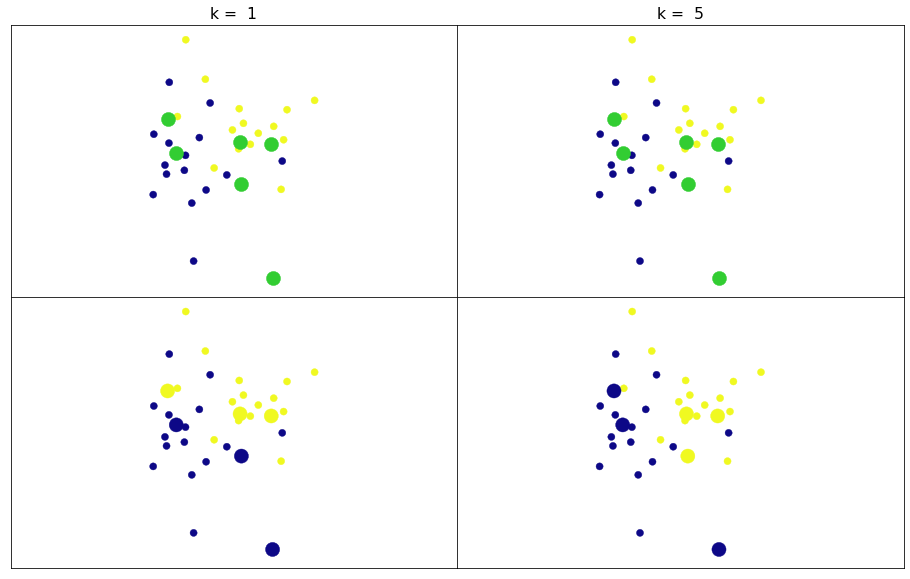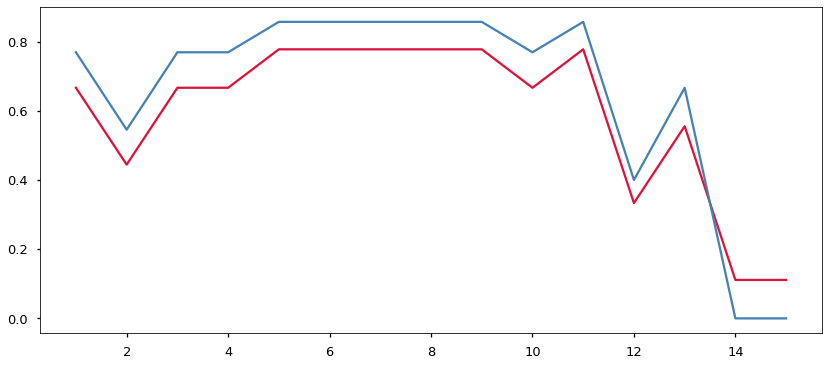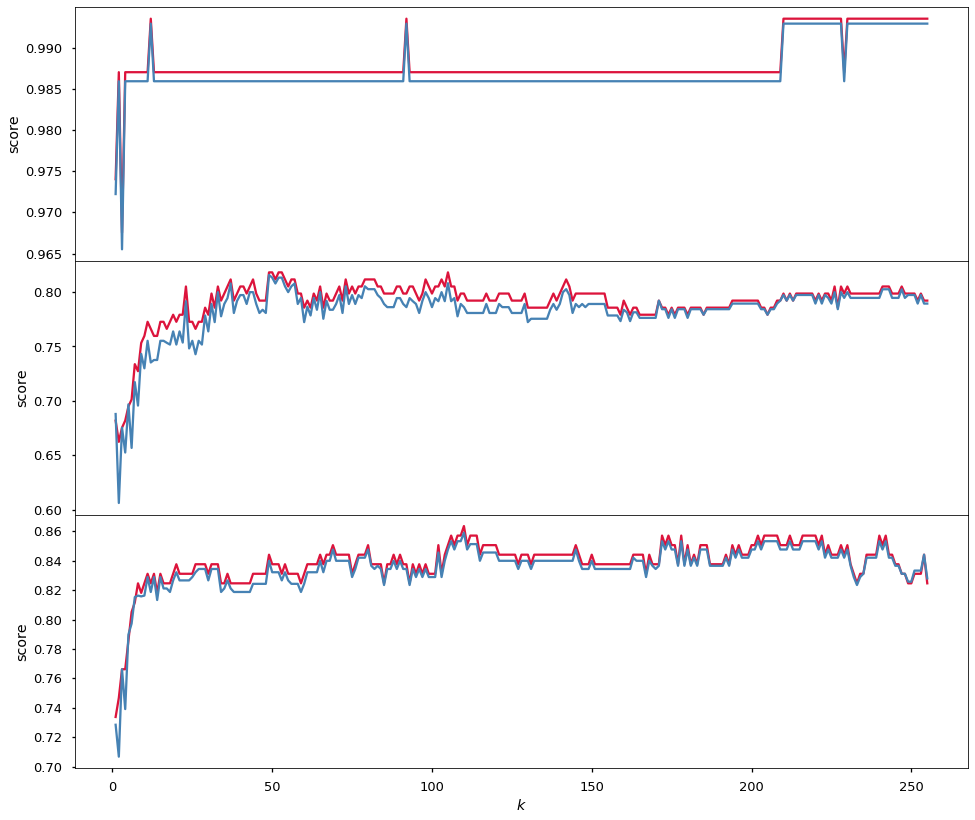# 06.03 - Is it right?¶

Writing a Machine Learning algorithm is only half the work. To use such an algorithm to produce a meaningful model and to be able to say that the predictions made by this model are likely correct is often an even harder job. Let us import a handful of useful things, we will go through the use of these procedures when we need them.skl-neighbours.svg
In :
import numpy as np
import matplotlib.pyplot as plt
%matplotlib inline
plt.style.use('seaborn-talk')
from sklearn.datasets import make_blobs
from sklearn.model_selection import train_test_split
from sklearn.metrics import accuracy_score, f1_score


We will also reconstruct our $k$ nearest neighbors algorithm. We know how the algorithm works hence we should take it for a ride.

In :
class MyKNN(object):
def __init__(self, k=1):
self.k_ = k

def fit(self, X, y):
self.X_ = X
self.y_ = y

def predict(self, new_X):
sq_dist_dim = (self.X_[:, np.newaxis, :] - new_X[np.newaxis, :, :])**2
sq_dist = sq_dist_dim.sum(axis=-1)
self.nearest_ = np.argpartition(sq_dist, self.k_, axis=0)
new_y = self.y_[self.nearest_[:self.k_, :]]
new_y = np.apply_along_axis(lambda x: np.bincount(x, minlength=2), axis=0, arr=new_y)
return new_y.argmax(axis=0)


The make_blobs procedure build a random dataset, a dataset that is within the limitations of our $k$ nearest neighbor implementation. We ask for $30$ points in $2$ dimensions, these will have $2$ centers: class $0$ which we will call blue, and class $1$ which we will call yellow. The randomness and spread of the blobs is fixed to generate one very specific dataset.

After that we generate $6$ more points, these we will call green. These new green points are data we have no knowledge whether they belong to the blue or yellow group. We will use our $k$ nearest neighbor algorithm to build a model that will predict the belonging of the green points.

Since $k$ is a hyperparameter - a free value - we can choose any value for it. We will choose $1$ and $5$ for $k$ and see how the results of classifying with these two models compare.

In :
random_state = 27
rng = np.random.RandomState(random_state)
X, y = make_blobs(30, 2, centers=2, cluster_std=0.2, center_box=(0, 1), random_state=random_state)
new_X = rng.rand(6, 2)

knn_left = MyKNN(k=1)
knn_left.fit(X, y)
new_yl = knn_left.predict(new_X)

knn_right = MyKNN(k=5)
knn_right.fit(X, y)
new_yr = knn_right.predict(new_X)

fig, ax = plt.subplots(2, 2, figsize=(16, 10))
ax[0, 0].set_title('k =  1')
ax[0, 0].scatter(X[:, 0], X[:, 1], s=50, c=y, cmap='plasma')
ax[0, 0].scatter(new_X[:, 0], new_X[:, 1], s=200, color='limegreen')
ax[1, 0].scatter(X[:, 0], X[:, 1], s=50, c=y, cmap='plasma')
ax[1, 0].scatter(new_X[:, 0], new_X[:, 1], s=200, c=new_yl, cmap='plasma')
ax[0, 1].set_title('k =  5')
ax[0, 1].scatter(X[:, 0], X[:, 1], s=50, c=y, cmap='plasma')
ax[0, 1].scatter(new_X[:, 0], new_X[:, 1], s=200, color='limegreen')
ax[1, 1].scatter(X[:, 0], X[:, 1], s=50, c=y, cmap='plasma')
ax[1, 1].scatter(new_X[:, 0], new_X[:, 1], s=200, c=new_yr, cmap='plasma')
for axi in ax.flat:
axi.axis('equal')
axi.set_xticks([], [])
axi.set_yticks([], [])
new_yl, new_yr

Out:
(array([0, 1, 1, 0, 0, 1]), array([0, 1, 0, 0, 1, 1]))On the left, where we used $k$ as $1$, we see northern green points classified as yellow and the southern green points as blue. On the right, where we used $k$ as $5$, we see the north-eastern green points classified as yellow and the south-western green points classified as blue. We also output the final classification made by our models for the green points, $0$ means a blue prediction and $1$ a yellow one.

The algorithm works but the difference in the classification when we change $k$ is considerable. Which of the models is right? Is any of them right? Is there a way for us to know which value of $k$ is more likely to produce good classification for the green points?

We cannot use the green points to check whether we are classifying them correctly because we do not know what they should be classified as in the first place. The only points we know the labels for are the points generated when building the blob. We must use the points for which we know the labels to validate out classifier (model). There are several ways to do it, let's start experimenting.

How NOT to do it:

In :
knn = MyKNN(k=1)
knn.fit(X, y)
new_y = knn.predict(X)
error = (y - new_y)**2
error.mean()

Out:
0.0

Here we train (fit) our model with $k$ as $1$. Then we predict the labels for each point. Finally we take the difference between what we predicted and what are the true labels. We square the differences so they are all positive and stake the mean. If there are no differences whatsoever the final result shall be zero. This is a simple form of an evaluation technique called appropriately: mean square error.

And the result for $k$ as $1$ is indeed zero. There have been no error in the predictions performed by our model. A perfect model! Which means $k$ as $5$ must perform worse, right?

In :
knn = MyKNN(k=5)
knn.fit(X, y)
new_y = knn.predict(X)
error = (y - new_y)**2
error.mean()

Out:
0.2

And yes, $k$ as $5$ performs with considerable error. There is something fishy about this. Can you tell?

When we used $k$ as $1$ each point we predicted has a closest point to itself in the training set at exactly $0$ distance - itself! One word used to describe such behavior is overfitting, and in this case it is maximum overfitting. We will come back to that concept eventually for now it is sufficient to say that: knowing that each point is closest to itself does not tell us anything about how that classification may help with figuring out the correct grouping for the green points.

To ask about the green points is to ask about data that our model has not been trained with, data that the model has not seen before. But we cannot use the greens since we do not have labels for them. Thankfully we could do a trick. We can train our model with a subset of the data from the set we know the labels for. After that, we will check the model prediction errors using the remaining data points for which we know the label for.

sklearn has an automated procedure for this. train_test_split divides a set of data, both features and labels, into a training and testing sets. The division happens at random, according to the ratio given in test_size. We will fix the random number generator for the time being.

To evaluate the performance of our models we will use the accuracy_score and f1_score procedures. These take the known labels and the predictions and output a value between $0$ and $1$, where (contrary to our manual attempts above) $1$ is the perfect score and $0$ the worse possible score. And since we have all this automation we will run the evaluation in a loop and attempt several more values for $k$.

In :
train_X, test_X, train_y, test_y = train_test_split(X, y, random_state=5, test_size=0.3)
accuracy = []
f1_scores = []
k_vals = list(range(1, 16))
for k in k_vals:
knn = MyKNN(k=k)
knn.fit(train_X, train_y)
new_y = knn.predict(test_X)
accuracy.append(accuracy_score(test_y, new_y))
f1_scores.append(f1_score(test_y, new_y))

fig, ax = plt.subplots(figsize=(14, 6))
ax.plot(k_vals, accuracy, color='crimson')
ax.plot(k_vals, f1_scores, color='steelblue');On this graph of $k$ values we can argue that a value of $5$ is indeed a good one. The smaller the value of $k$ the faster the model runs, hence our decision should be for the smallest value which still gives a good score. That said, the purpose here is illustrative. In our set of $30$ points here are more differences as to what $k$ we would choose based on how the data is split into training and testing set. In this case if we change the value to which we fix the random number generator we will have a rather different graph. This is caused because we have rather few data points to choose from, a dataset of $30$ points is just too small.

Let's hence see how this all works on a bigger set. And we should try it a couple of times to see if we see any patterns. We will now make the blobs with $512$ points instead of $30$. We will try out values for $k$ from $1$ up to $255$. And we will repeat the procedure $3$ times, each time we generate a different blob but with similar characteristics.

In :
def select_k(ax):
X, y = make_blobs(512, 2, centers=2, cluster_std=0.2, center_box=(0, 1))
train_X, test_X, train_y, test_y = train_test_split(X, y, test_size=0.3)
accuracy = []
f1_scores = []
k_vals = list(range(1, 256))
for k in k_vals:
knn = MyKNN(k=k)
knn.fit(train_X, train_y)
new_y = knn.predict(test_X)
accuracy.append(accuracy_score(test_y, new_y))
f1_scores.append(f1_score(test_y, new_y))
ax.plot(k_vals, accuracy, color='crimson')
ax.plot(k_vals, f1_scores, color='steelblue')
ax.set(xlabel='$k$', ylabel='score')

fig, ax = plt.subplots(3, 1, figsize=(16, 14), sharex=True)The graphs do look different from each other indeed. Although we can see a pattern for a good value for $k$ emerging. Certainly for any set of blobs with around this number of points and this standard deviation a good value of $k$ would be below $50$.